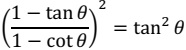# ICSE Class 10 Mathematics 2020 Question Paper

The duration of the paper is two hours and a half. Attempt all questions from Section A and any four questions from Section B.

### Section A (40 Marks)

Question 1

(a) Solve the following Quadratic Equation: x2 - 7𝑥 + 3 = 0

(b) Given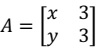If A2 = 3I, where I is the identity matrix of order 2, find x and y. 

(c) Using ruler and compass construct a triangle ABC where AB = 3 cm, BC = 4 cm and ∠ABC = 90º. Hence construct a circle circumscribing the triangle ABC. Measure and write down the radius of the circle. 

Question 2

(a) Use factor theorem to factorise 6x3 + 17x2 + 4x - 12 completely. 

(b) Solve the following inequation and represent the solution set on the number line.

3x/5 + 2 < 𝑥 + 4 ≤ x/2 + 5, x ∈ 𝑅 

(c) Draw a Histogram for the given data, using a graph paper: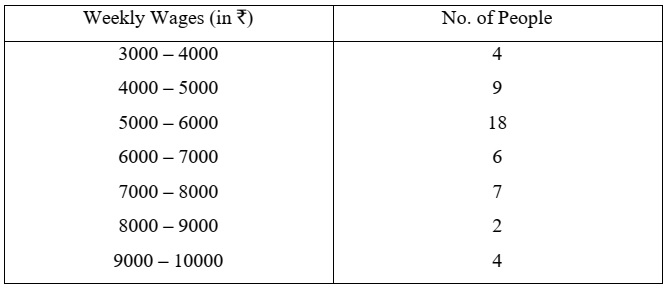Estimate the mode from the graph. 

Question 3

(a) In the figure given below, O is the centre of the circle and AB is a diameter.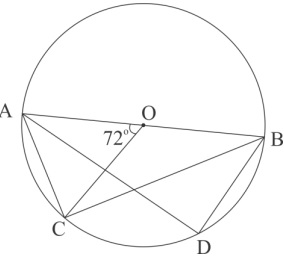If AC = BD and ∠AOC = 72º. Find:

1. ∠ABC
3. ∠ABD 

(b) Prove that: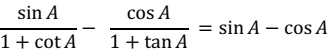(c) In what ratio is the line joining P(5, 3) and Q(-5, 3) divided by the y-axis? Also find the coordinates of the point of intersection. 

Question 4

(a) A solid spherical ball of radius 6 cm is melted and recast into 64 identical spherical marbles. Find the radius of each marble. 

(b) Each of the letters of the word ‘AUTHORIZES’ is written on identical circular discs and put in a bag. They are well shuffled. If a disc is drawn at random from the bag, what is the probability that the letter is:

1. a vowel
2. one of the first 9 letters of the English alphabet which appears in the given word
3. one of the last 9 letters of the English alphabet which appears in the given word? 

(c) Mr. Bedi visits the market and buys the following articles:

Medicines costing ₹ 950, GST @ 5%
A pair of shoes costing ₹ 3000, GST @ 18%
Laptop bag costing ₹ 1000 with a discount of 30%, GST @ 18%.

1. Calculate the total amount of GST paid.
2. The total bill amount including GST paid by Mr. Bedi. 

### Section B (40 Marks)

Question 5

(a) A company with 500 shares of nominal value ₹ 120 declares an annual dividend of 15%. Calculate:

1. the total amount of dividend paid by the company.
2. annual income of Mr. Sharma who holds 80 shares of the company.

If the return percent of Mr. Sharma from his shares is 10%, find the market value of each share. 

(b) The mean of the following data is 16. Calculate the value of f.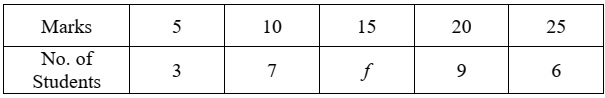(c) The 4th, 6th and the last term of a geometric progression are 10, 40 and 640 respectively. If the common ratio is positive, find the first term, common ratio and the number of terms of the series. 

Question 6

(a) If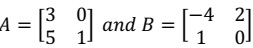Find A2 - 2AB + B2 

(b) In the given figure AB = 9 cm, PA = 7.5 cm and PC = 5 cm. Chords AD and BC intersect at P.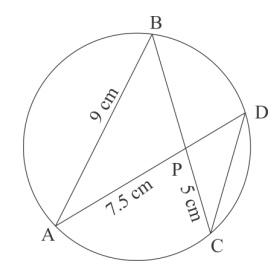1. Prove that ∆PAB ~ ∆PCD
2. Find the length of CD.
3. Find area of ∆PAB : area of ∆PCD 

(c) From the top of a cliff, the angle of depression of the top and bottom of a tower are observed to be 45° and 60° respectively. If the height of the tower is 20 m. Find:

1. the height of the cliff
2. the distance between the cliff and the tower. 

Question 7

(a) Find the value of 'p' if the lines, 5x - 3y + 2 = 0 and 6x - py + 7 = 0 are perpendicular to each other. Hence find the equation of a line passing through (-2, -1) and parallel to 6x - py + 7 = 0. 

(b) Using properties of proportion find x ∶ y, given:

(x2 + 2x)/(2x + 4) = (y2 + 3y)/(3y + 9) 

(c) In the given figure TP and TQ are two tangents to the circle with centre O, touching at A and C respectively.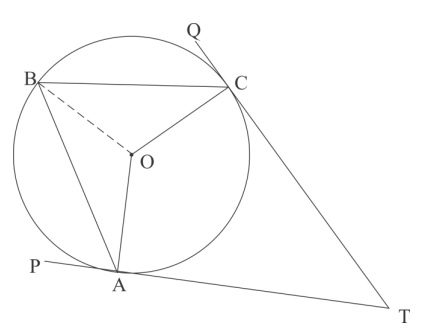If ∠BCQ = 55° and ∠BAP = 60o, find:

1. ∠OBA and ∠OBC
2. ∠AOC
3. ∠ATC 

Question 8

(a) What must be added to the polynomial 2x3 - 3x2 - 8x, so that it leaves a remainder 10 when divided by 2x + 1? 

(b) Mr.Sonu has a recurring deposit account and deposits ₹ 750 per month for 2 years. If he gets ₹ 19125 at the time of maturity, find the rate of interest. 

(c) Use graph paper for this question. Take 1 cm = 1 unit on both x and y axes.

(i) Plot the following points on your graph sheets:

A(-4, 0), B(-3, 2), C(0, 4), D(4, 1) and E(7, 3)

(ii) Reflect the points B, C, D and E on the x-axis and name them as B', C', D' and E' respectively.

(iii) Join the points A, B, C, D, E, E', D', C', B' and A in order.

(iv) Name the closed figure formed. 

Question 9

(a) 40 students enter for a game of shot-put competition. The distance thrown (in metres) is recorded below: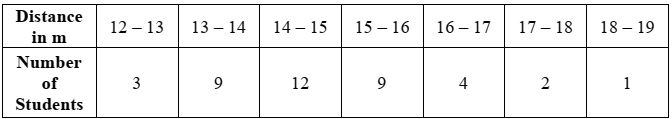Use a graph paper to draw an ogive for the above distribution.

Use a scale of 2 cm = 1 m on one axis and 2 cm = 5 students on the other axis.

1. the median
2. Upper Quartile
3. Number of students who cover a distance which is above 16½ m. 

(b) If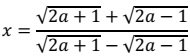, prove that x2 - 4ax + 1 = 0 

Question 10

(a) If the 6th term of an A.P. is equal to four times its first term and the sum of first six terms is 75, find the first term and the common difference. 

(b) The difference of two natural numbers is 7 and their product is 450. Find the numbers. 

(c) Use ruler and compass for this question. Construct a circle of radius 4.5 cm. Draw a chord. AB = 6 cm.

1. Find the locus of points equidistant from A and B. Mark the point where it meets the circle as D.
2. Join AD and find the locus of points which are equidistant from AD and AB. Mark the point where it meets the circle as C.
3. Join BC and CD. Measure and write down the length of side CD of the quadrilateral ABCD. 

Question 11

(a) A model of a high rise building is made to a scale of 1 : 50.

1. If the height of the model is 0.8 m, find the height of the actual building.
2. If the floor area of a flat in the building is 20 m2, find the floor area of that in the model. 

(b) From a solid wooden cylinder of height 28 cm and diameter 6 cm, two conical cavities are hollowed out. The diameters of the cones are also of 6 cm and height 10.5 cm.

Taking π = 22/7, find the volume of the remaining solid.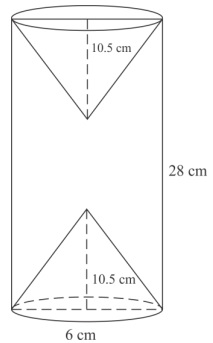(c) Prove the identity